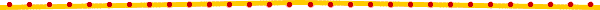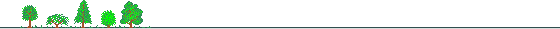# Chapter 2Calculating Formulas of the Rates of Return## 2.1 Daily Rate of Return of Common Stocks

The daily rate of return of a common stock for the days when there is neither ex-cash dividend nor ex-right:

 Daily Rate of Return = [(Today's Closing Price / Previous Trading Day's Closing Pirce) - 1] x 100

The daily rate of return of a common stock for the days when there is either ex-cash dividend or ex-right:

 Daily Rate of Return = {[Today's Closing Price x (1+S%+N%) + Cash Divident] / (Previous Trading Day's Closing Pirce + FxN%) - 1} x 100

 where S% = Stock Dividend Percentage; N% = New Issue Percentage; F = Flotation Price of New Issue.

## 2.2 Daily Rate of Return of Preferred Stocks

The daily rate of return of a preferred stock for the days when there is neither ex-cash dividend nor ex-right:

 Daily Rate of Return = [(Today's Closing Price / Previous Trading Day's Closing Pirce) - 1] x 100

The daily rate of return of a preferred stock for the days when there is either ex-cash dividend or ex-right:

 Daily Rate of Return = {[Preferred Stock's Closing Price + Common Stock's Closing Price x (S% + N%) + Cash Dividend] / (Preferred Stock's Previous Trading Day's Closing Price + F x N%) - 1} x 100

 where S%, N%, F are as defined above.

## Weekly, Monthly, Quarterly and Annual Rates of Return

The weekly rate of return is calculated as follows:

 Weekly Rate of Return = (1 + Daily Rate of Return on the First Trading Day of the Week) x (1 + Daily Rate of Return on the 2nd Trading Day of the Week) x ....... x (1 + Daily Rate of Return on the Last Trading Day of the Week) - 1

The formulas for the monthly, quarterly and annual rates of return are similar to the formula for the weekly rate of return above.